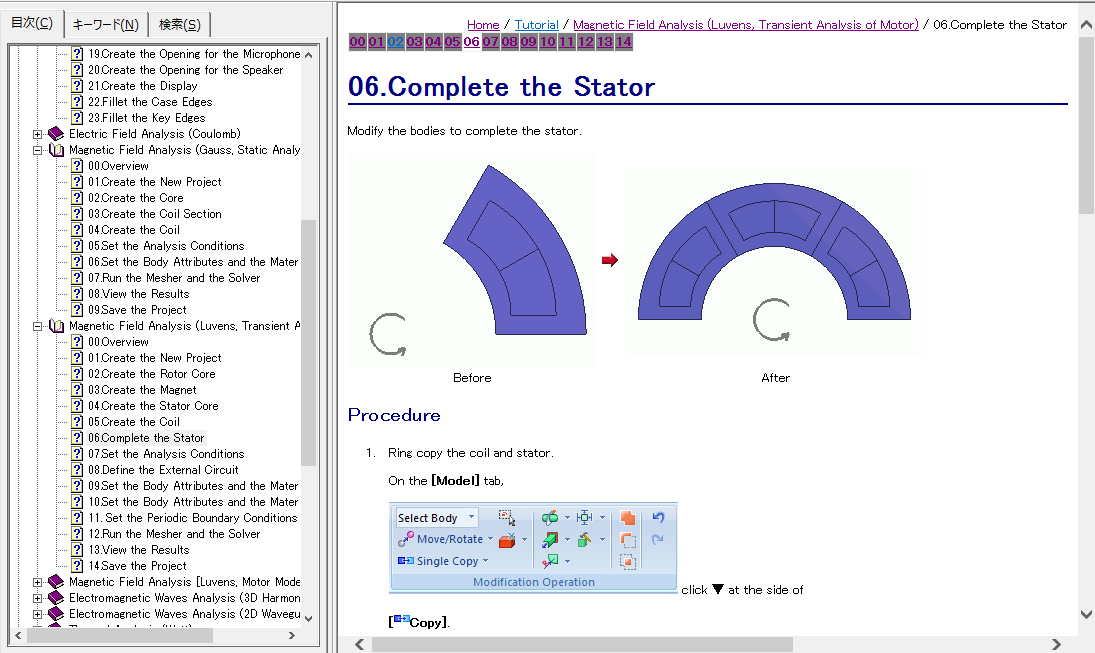﻿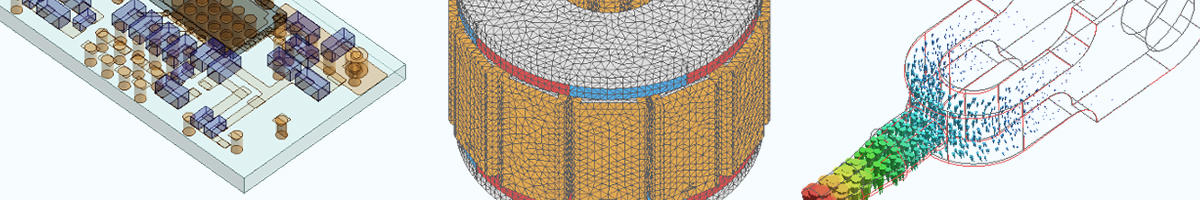# Femtet at a Glance

Femtet is all-in-one CAE software equipped with 3D solid modeler, pre-/post-processors and 8 types of solvers.
Tutorials and examples too are available. Here is a brief look at each functionality.

## Modeling / Results Display

Femtet allows intuitive operations of modeling without knowing complicated commands in depth. Import and export of CAD data are supported.

Meshing

Triangle or tetrahedral meshes are automatically generated by simply pressing a button. Partial mesh size setting and adaptive meshing are supported as well. Mesh size suitable for individual analysis is set automatically. Femtet can import mesh data in Nastran format for analysis.

Results Display

Simulation results are displayed in various ways like contour diagram, deformation diagram, vector diagram, cross-sectional diagram, and animation. The results can be saved in csv format.

Modeling Window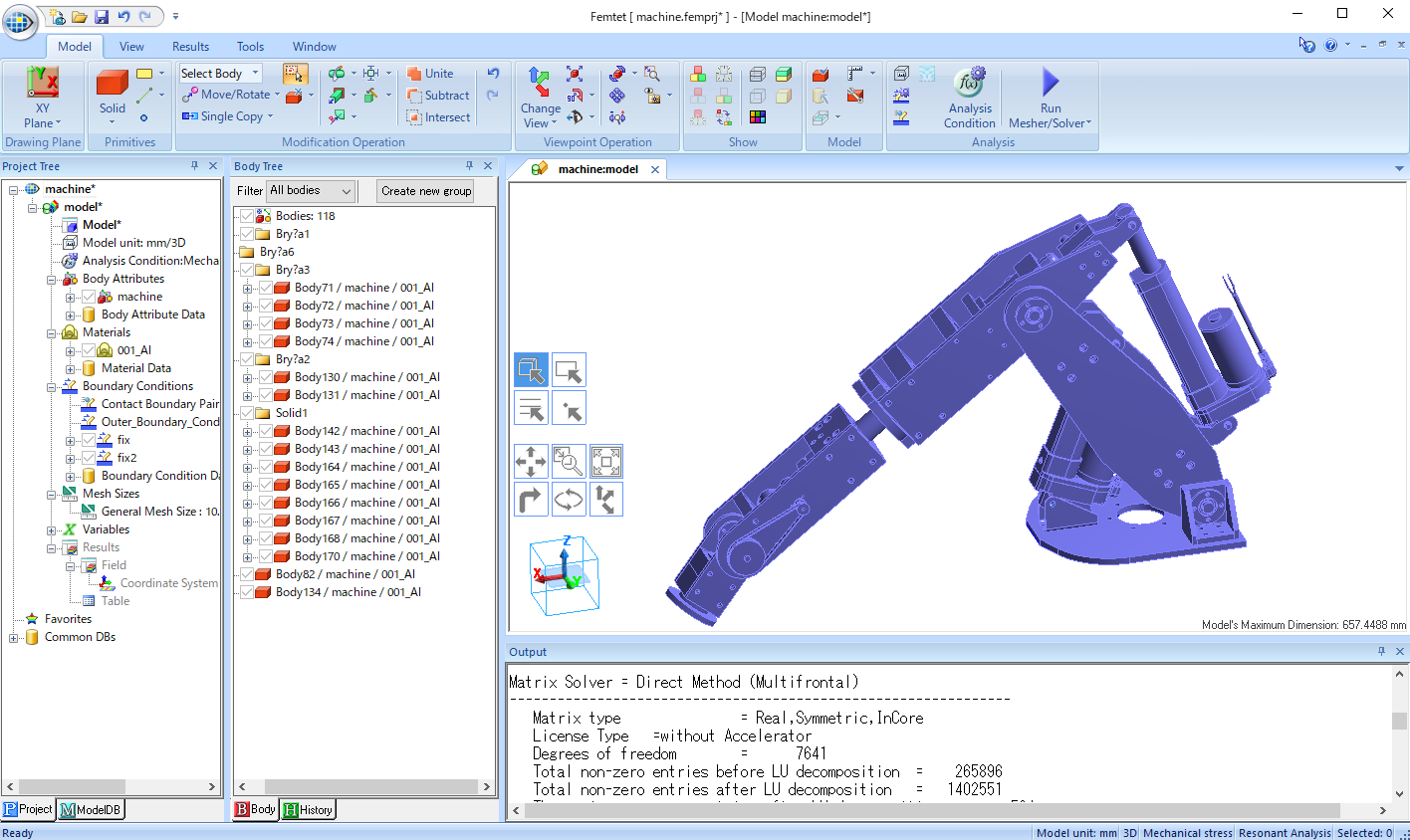Results Window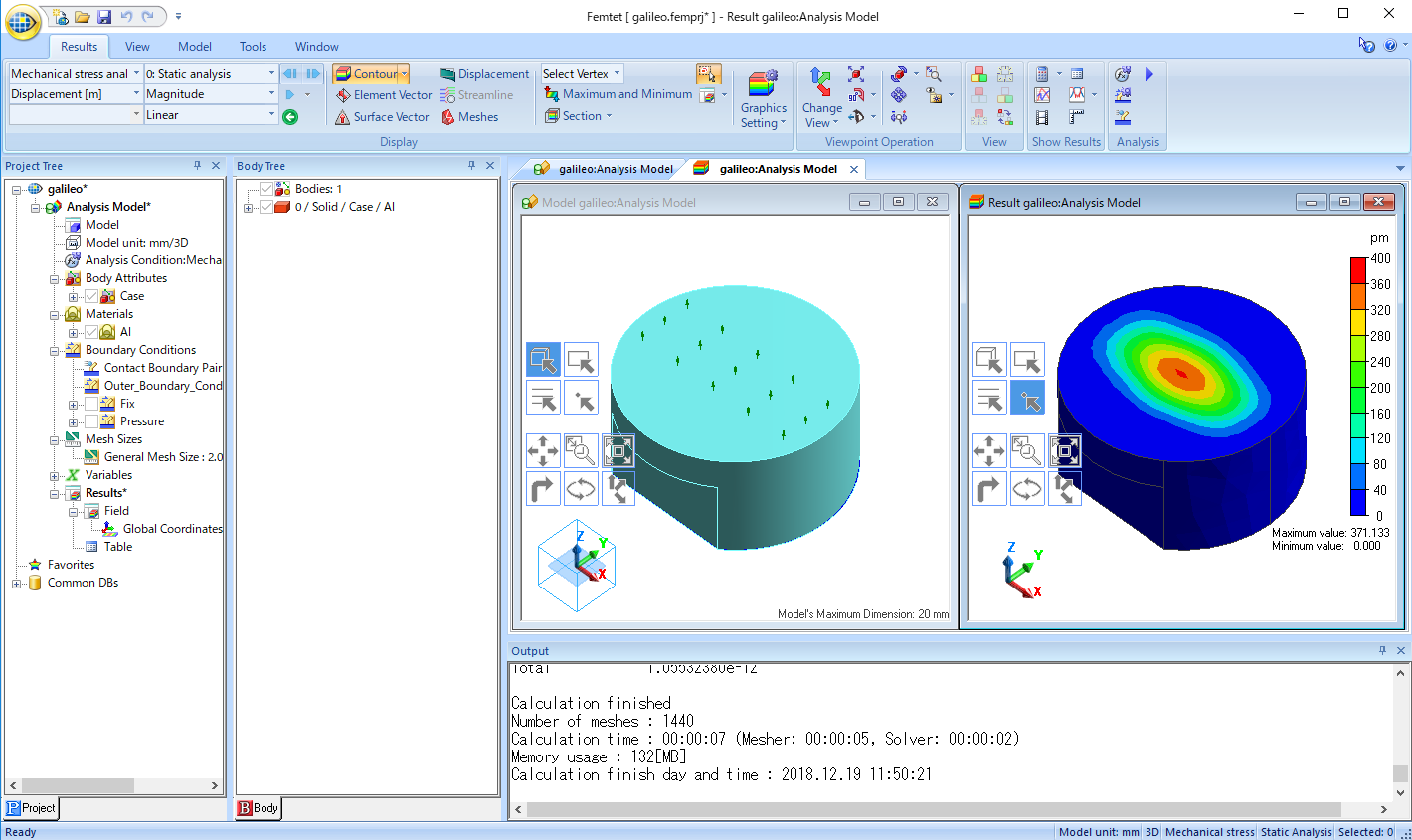## Electromagnetic Analysis

Propagation constant, S-, Y-, Z-parameters, radiation characteristics, and magnetic field distribution are solved. Differential transmission line and TDR are calculated as well. Saving data in the Touchstone format allows analysis with a circuit simulator.

Antenna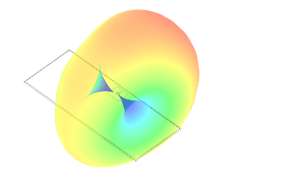Wireless Power Transmission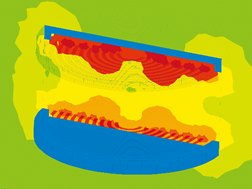TDR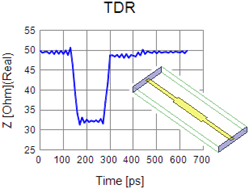## Magnetic Analysis

Magnetic field distribution, magnetic flux density, induced current, and magnetic force are solved. Also, inductance and impedance characteristics, coupling coefficient, loss, and motor characteristics (torque, T-N, T-I) are solved. A transient analysis is available to solve problem like arbitrary waveform. Materials with nonlinear B-H curve are calculated as well.

Solenoid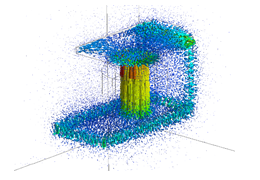Transformer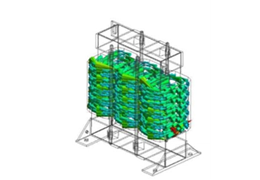Motor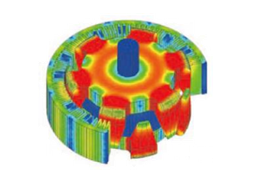## Electric Analysis

Electric field and current distributions of dielectric and conductive materials with voltage being applied are solved. Also, capacitance and resistance across electrodes and force applied to dielectric material are solved. Plating and hall element are calculated as well.

Touchscreen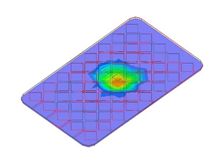Resistance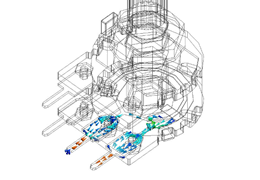Plating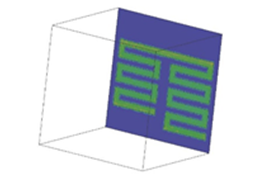## Stress Analysis

Mechanical stress, displacement, and deformation are solved. Problems like structural behavior under load acceleration, bending by self weight are calculated. Deformation by thermal load and impact on a falling object are analyzed as well. Elastic, elasto-plastic, viscoelastic, and hyperelastic materials are analyzed.

Contact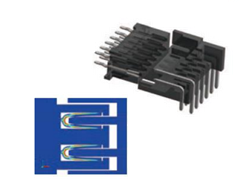Resonance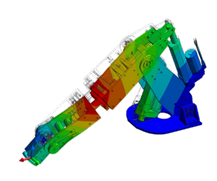Life of Solder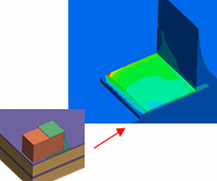## Thermal Analysis

Heat conductivity, heat transfer, radiation, and heat flux are solved. Steady-state analysis and transient analysis together with nonlinear material will realize the optimum thermal design. Simple-fluid thermal analysis is available as well.

Heat Transfer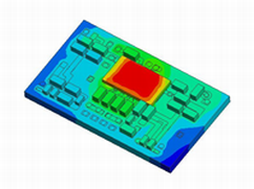Heat Dissipation of LED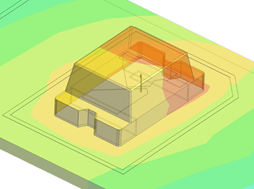Air Cooling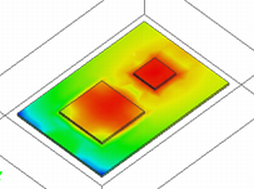## Fluid Analysis

Flows of liquid and air are analyzed. The flows with an obstacle in the flow path can be calculated as well.
Forced convection can be analyzed by coupling with thermal analysis.

Fluid Velocity of the Air around an Automobile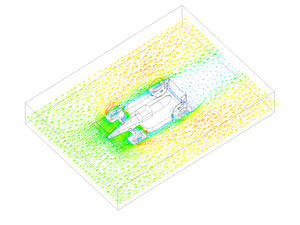Fluid Velocity around an Obstacle in the Flow Path (Displayed in the Streamlines)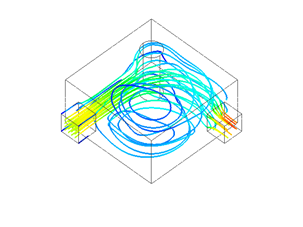Heat Cooling of the Substrate (Coupled Analysis with Thermal Solver)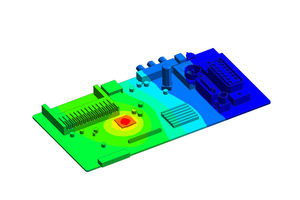## Piezoelectric Analysis

Structures made of quartz crystal or piezoelectric ceramic are analyzed. Static analysis, Eigen value analysis, frequency characteristics analysis, and transient analysis are available. Vibration distribution and impedance characteristics are solved for devices like sensor, buzzer, and actuator.

Horn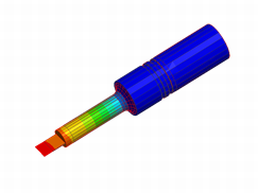Piezoelectric Gyrosensor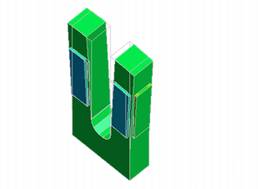Ultrasonic Motor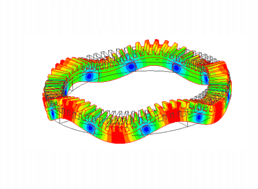## Acoustic Analysis

Sound pressure distribution and particle velocity distribution are solved. Reflection, diffraction, resonance and directivity of sound waves are calculated as well. Coupling analysis with piezoelectric solver allows calculation of sound waves propagation generated by a vibrating device like back sonar.

Speaker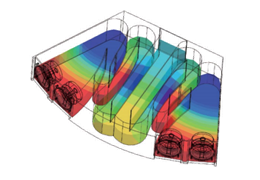Ultrasonic Sensor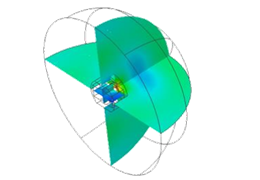Reflection & Diffraction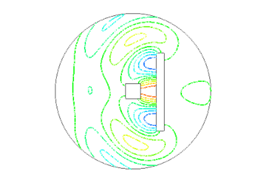## Macros

Macros allow advanced analysis, such as calculation while changing dimensions, giving frequency dependency to materials, and integration with other tools in the market.

Example: Macro Programming (VBA)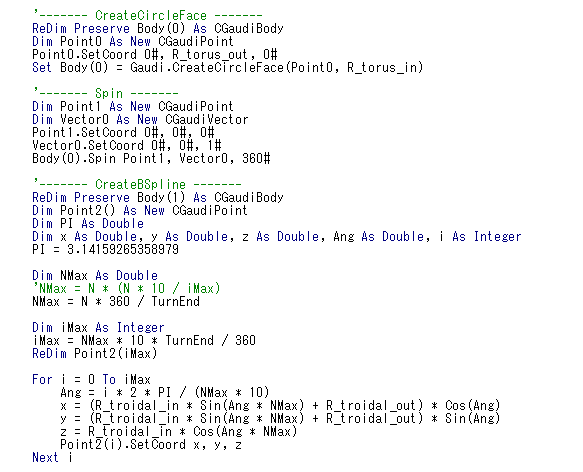## Tutorials / Examples

Learn and familiarize yourself with Femtet operations.

Tutorials